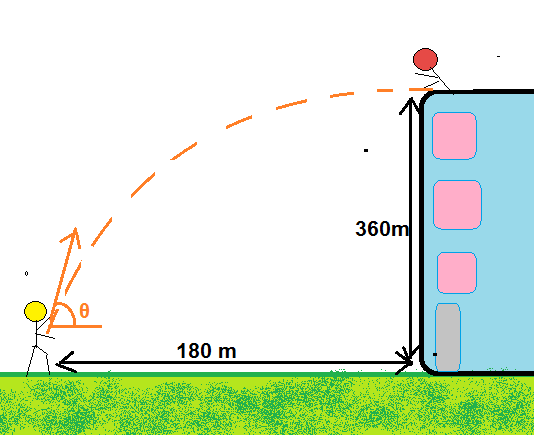# Chocolate mania (mechanics +trigonometry)Cody is this time throwing a chocolate from the ground and the $\color{#D61F06}{\text{lady}}$ is on the top of the building to catch it.

The point from where Cody throws the chocolate is $180\, \text{m}$ away from the building and building is $360\,\text{m}$ tall.

Given that when the $\color{#D61F06}{\text{lady}}$ caught the chocolate, it was travelling horizontally (vertical velocity $0\,\text{m/s}$) and Cody had thrown the chocolate with the initial velocity making an angle $\theta$ with the horizontal, then find the value of

$\tan \theta \times \tan (60^\circ -\theta) \times \tan(60^\circ +\theta)$

This value can be written as $\dfrac{a}{b}$ for coprime positive integers $a$ and $b$ . then find the value of $a+b$.

Details: In this problem, you don't need the initial velocity at all, neither the value of acceleration due to gravity.

×